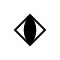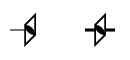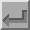Fourfold Inversion Axis (-4)The symbol above shows a fourfold inversion axis, i.e. a fourfold inversion axis perpendicular to the screen. A fractional value next to the symbol indicates the height of the inversion centre above the XY plane. As with the fourfold axis, a positive rotation is anticlockwise. A fourfold rotation about the c-axis, i.e. about the line 0,0,z, followed by an inversion at the origin will have the corresponding symmetry operator y,-x,-z. This axis has the written symbol "-4" (where the minus sign is usually written above the digit). The solid centre of the symbol emphasises the implied twofold axis that results from a fourfold inversion axis.In space group diagrams for cubic symmetry, fourfold inversion axes are shown along both X and Y directions as well as along the Z direction when present. These are shown by the symbols above. The right-hand symbol shows the inversion point along the fourfold inversion axis. By constrast, the line in the left-hand symbol points towards the inversion point. A fractional value next to either symbol indicates the height of the axis of rotation above the XY plane.Back
 © Copyright 1995-2006.  Birkbeck College, University of London. Author(s): Jeremy Karl Cockcroft